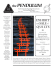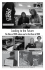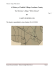# Ex8 - Informatik

## Transcription

Ex8 - Informatik
```CS331: Machine Learning
Prof. Dr. Volker Roth
[email protected]
FS 2015
Aleksander Wieczorek
[email protected]
Dept. of Mathematics and Computer Science
Spiegelgasse 1
4051 Basel
Date: Monday, May 4th 2015
Exercise 11: Kernel ridge regression
Recap & Definitions
• Ridge regression:
– The problem is formulated as:
p
p
n
X
X
X
(yi − β0 −
βj gj (xi ))2 + ν
βj2
βˆridge = argminβ
i=1
j=1
!
,
j=1
where ν ∈ [0, ∞) is a penalty parameter.
– In matrix form:
βˆridge = argminβ (y − Xβ)t (y − Xβ) + νβ t β .
– The solution is given by:
βˆridge (X t X − νI) = X t y
and using the SVD decomposition of X we have:
βˆridge = V (S t S + νI)−1 S t U t y
• Kernel ridge regression:
– Expand β in terms of input vectors: β = X t α, α ∈ Rp+1 .
– The problem is then reformulated as:
α
ˆ ridge = argminα (y − XX t α)t (y − XX t α) + ναt XX t α .
– Substitute the dot product matrix XX t by an arbitrary Mercer-kernel matrix K:
α
ˆ ridge = argminα (y − Kα)t (y − Kα) + ναt Kα .
– Setting the derivative ∂/∂α to zero gives:
2K t (Kα − y + να) = 0
and the solution is given by:
α
ˆ ridge (K + νI) = y
• Predictions based on a linear combination of a set of radial basis functions:
1
gi (x) = K(x, xi ) = exp − k x − xi k2 , xi , x ∈ Rd ,
λ
and λ ∈ (0, ∞) is the smoothing parameter.
1
CS331: Machine Learning
FS 2015
Exercise
Write a Matlab function for kernel ridge regression with RBF and x ∈ R. Allow an intercept in
the model, i.e. add 1 to each element of the kernel matrix. With the optimal α
ˆ ridge calculated
on training data X make predictions for new test data x? : yˆ? = K(x? , x)α
ˆ ridge and compute
the test set error. (Generate data as in exercise sheet 5 and compare.)
function [err,model,errT] = KridgeRFB(x,y,lambda,nu,xT,yT)
x = vector of input scalars for training
y = vector of output scalars for training
lambda = RBF width parameter (>0)
nu = ridge penalty (>=0)
xT = vector of input scalars for testing
yT = vector of output scalars for testing
err = average squared loss on training
alphaHat = vector of parameters
errT = average squared loss on testing
2
```

### Manaslu East Ridge Attempt. Hervé Thivierge, Gilles Claret Tournier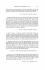### Dial-a-Ride service area expanding July 6, 2015.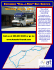### South Ridge of Teepe`s Pillar. Willi and Jolene Unsoeld and Norman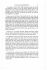### ÅSBRORUNDAN, 18 km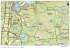### see brochure preview here - vareal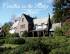### Name - MerchantsOfOakRidge.com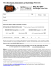### The Chase at Overlook Ridge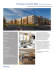### Read Across America, Drop-off Safety, Make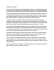### For Technical Assistance Contact: OMIX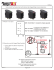### Lot backing up to Open Space - Taverner Ridge Estates | Custom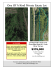### FOR IMMEDIATE RELEASE Contact: Holly Rood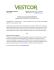### WANT BUTTERFLIES? DON`T FORGET THE CATERPILLARS!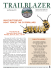### Winter 2015 issue - Pound Ridge Historical Society